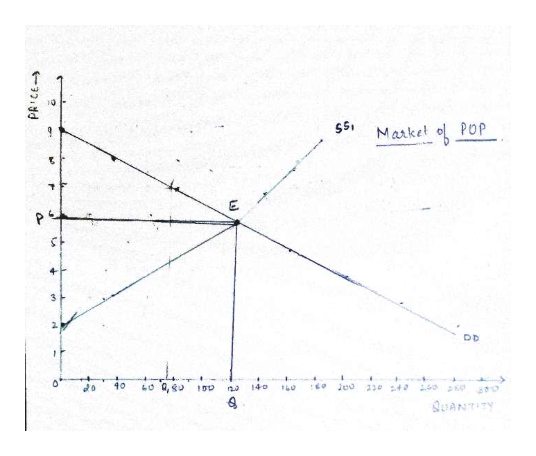# 1. The demand and supply schedules for pop in Vancouver are as follows: Price (\$/pack of 2 bottles) Quantity demanded (thousands /week) Quantity supplied (thousands/ week) 2 280 0 3 240 30 4 200 60 5 160 90 6 120 120 7 80 140 8 40 160 9 0 180 a. (1) With the use of a demand and supply diagram, show the market equilibrium. b. (2) Now suppose that a fire destroys one-half of the pop producing factories. Supply decreases to one half shown in the above supply schedule. What are the new equilibrium price and quantity of pop? Show the new equilibrium in your diagram from part a). c. (1) Has there been a shift or a movement along the supply curve of pop in part b)? d. (2) As the pop factories destroyed by fire are rebuilt and gradually resume pop production, what will happen to the price of pop? The quantity of pop bought? The demand curve for pop?

Question
62 views

1. The demand and supply schedules for pop in Vancouver are as follows: Price (\$/pack of 2 bottles) Quantity demanded (thousands /week) Quantity supplied (thousands/ week) 2 280 0 3 240 30 4 200 60 5 160 90 6 120 120 7 80 140 8 40 160 9 0 180 a. (1) With the use of a demand and supply diagram, show the market equilibrium. b. (2) Now suppose that a fire destroys one-half of the pop producing factories. Supply decreases to one half shown in the above supply schedule. What are the new equilibrium price and quantity of pop? Show the new equilibrium in your diagram from part a). c. (1) Has there been a shift or a movement along the supply curve of pop in part b)? d. (2) As the pop factories destroyed by fire are rebuilt and gradually resume pop production, what will happen to the price of pop? The quantity of pop bought? The demand curve for pop?

check_circle

Step 1

a. (1) The diagram below shows the pop market with DD as the demand curve and SS1 as the supply curve. Both the curve intersects each other at point E where the equilibrium quantity is 120 units whereas the equilibrium price is \$6.help_outlineImage TranscriptionclosePOP Market P 4 3 DD 40 40260aoo Jeo 140 230 40 QUANTITY 32dd fullscreen
Step 2

b. (2) One-half reduction of supply in the market due to fire leads to a new supply curve that is shown in the diagram below. A new supply curve SS1 will intersect the DD curve at new equilibrium point E1 where equilibrium quantity is Q1 that is equal to 75 units and equilibrium price is P1 which is equal to \$7.25 from the graph.

Step 3

c. (1) Fire that leads to a reduction in supply to one half leads to a shift in the sup...

### Want to see the full answer?

See Solution

#### Want to see this answer and more?

Solutions are written by subject experts who are available 24/7. Questions are typically answered within 1 hour.*

See Solution
*Response times may vary by subject and question.
Tagged in